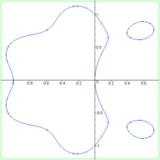Polynomial Lemniscate

# Polynomial lemniscate

to get instant updates about 'Polynomial Lemniscate' on your MyPage. Meet other similar minded people. Its Free!

X

Description:
In mathematics, a polynomial lemniscate or polynomial level curve is a plane algebraic curve of degree 2n, constructed from a polynomial p with complex coefficients of degree n.

For any such polynomial p and positive real number c, we may define a set of complex numbers by [itex]|p(z)| = c.[/itex] This set of numbers may be equated to points in the real Cartesian plane, leading to an algebraic curve ƒ(x,&nbsp;y)&nbsp;=&nbsp;c<sup>2</sup> of degree 2n, which results from expanding out [itex]p(z) bar p(bar z)[/itex] in terms of z&nbsp;=&nbsp;x&nbsp;+&nbsp;iy.

When p is a polynomial of degree 1 then the resulting curve is simply a circle whose center is the zero of p. When p is a polynomial of degree 2 then the curve is a Cassini oval.

## Erdős lemniscate

A conjecture of Erdős which has attracted considerable interest concerns the maximum length of a polynomial lemniscate &fnof;(x,&nbsp;y)&nbsp;=&nbsp;1 of degree 2n when p is monic, which Erdős conjectured was attained when p(z)&nbsp;=&nbsp;z<sup>n</sup>&nbsp;&minus;&nbsp;1. In the case when n&nbsp;=&nbsp;2, the Erdős lemniscate is the Lemniscate of Bernoulli

[itex](x^2+y^2)^2=2(x^2-y^2),[/itex]

and it has been proven that this is indeed the maximal length in degree four. The Erdős lemniscate has three ordinary n-fold points, one of which is at the origin, and a genus of...

No feeds found

AllPosting your question. Please wait!...